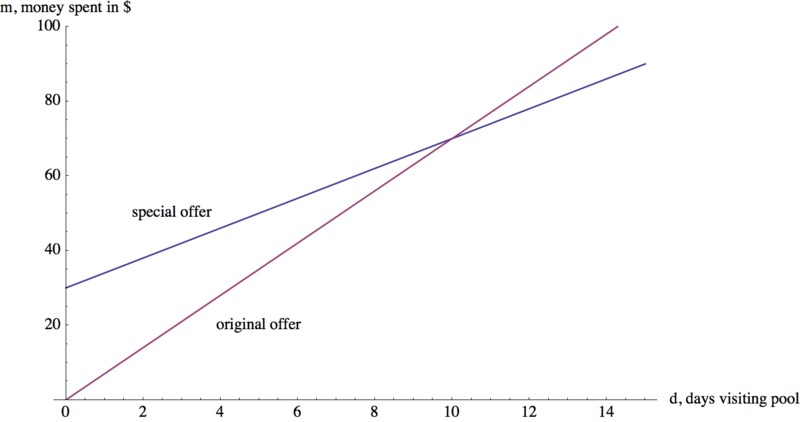# Summer Swimming

Alignments to Content Standards: 8.EE.C.8.c

The local swim center is making a special offer. They usually charge \$7 per day to swim at the pool. This month swimmers can pay an enrollment fee of \$30 and then the daily pass will only be \$4 per day. 1. Suppose you do not take the special offer. Write an equation that represents the amount of money you would spend based on how many days you go to the pool if the passes were bought at full price. 2. Write a second equation that represents the amount of money you would spend if you decided to take the special offer. 3. Graph your two equations from part (a) and (b). 4. After how many days of visiting the pool will the special offer be a better deal? How can you tell algebraically? How can you see this graphically? 5. You only have \$60 to spend for the summer on visiting this pool. Which offer would you take? Explain.

## IM Commentary

The purpose of this task is for students to represent relationships between quantities in a context with equations and interpret the resulting system of equations in the context. This task has a wide array of uses: it could be an introductory task to systems of equations or used in assessment. The task initially has the students write out different equations for two different scenarios. Then the students can consider the two equations together, which naturally leads into a discussion on the point of intersection of two graphs and into systems of equations. Students are asked to reason abstractly and make sense of the representations of the relationships between two quantities in the context (MP2).

This task also asks the student to not only consider what $m$ (the amount of money they spend) would be for different values of $d$ (the number of days they swim) but what would $d$ be for a certain value of $m$. Although it is not mentioned in the solution until part (e), this task also provides a good opportunity to discuss the fact that only integer values of $d$ make sense in this context, although we make a continuous line in our graphs. We do this to see the general trend and compare the two relationships more easily, and because we are asked to graph our equations, not a table of discrete values.

## Solution

1. Let $m$ represent the amount of money you spend, and let $d$ represent the number of days you go to the pool. At full price, you would spend \$7 per day, so$m=7d$represents the amount of money you would spend without the special offer. 2. Again, let$m$represent the amount of money you spend, and let$d$represent the number of days you go to the pool. With the special offer, you would spend \$30 once, and then \$4 per day, so$m=4d+30$represents the amount of money you would spend with the special offer. 3. We can graph these equations on the same set of axes:4. When considering when the special offer will become a better deal, we want to find out after how many days we will be spending less money than with the full price passes. For example, after 3 days, the full price offer will cost us \$21 dollars and the special offer will cost us \$39, so the special will cost more. We see that this is because of the initial fee of \$30.

Algebraically, we can determine exactly when the special offer costs the same amount as the standard price by setting our two expressions for $m$ equal to each other and solving for $d$. $$4d+30=7d$$ $$30=3d$$ $$d=10.$$ So, when $d=10$, or on the 10th day, the special offer and the original will have cost us the same amount of money: $$m=7(10)=70$$ and $$m=4(10)+30=70.$$ Because on the 11th day, and every day thereafter, the special offer will cost less (\$4 a day versus \$7 a day), we can conclude that after the 10th day is when the special offer becomes a better deal.

Graphically, we can see that the graph of the special offer drops below the graph of the original offer after $d=10$, and as lower translates into less money spent, we can see our conclusion in the graph, as well.

5. We have a total of \$60 to spend, and so$m=60$. We can substitute this into the equation for each offer. Special offer: $$60=4d+30 \implies 30=4d \implies \frac{30}{4}=7.5=d$$ Since$d$represents days, and we can only go for full days,$.5$of a day does not make sense, and so we find that with \$60, we can go to the pool for 7 days if we take the special offer.

Original offer: $$60=7d \implies \frac{60}{7}=8\frac{4}{7}=d$$ Again, we cannot visit the swim center for $\frac{4}{7}$ of a day, and so with \$60 we are able to go for a full 8 days if we take the full price offer. Therefore, we should take the original offer if we only have \$60 to spend, because we can go for an additional day over the special offer.